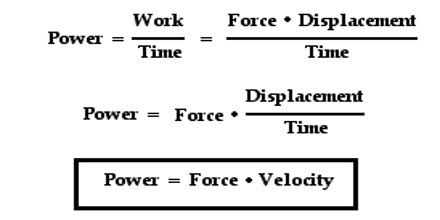Physics

# Unit of PowerUnit of power

Power is the rate at which work is done or energy is transferred in a unit of time. It is increased if work is done faster or energy is transferred in less time.

From the definition of power, its unit can be found out.

Power = work/time = Joule/sec ( Js-1)

Dimension: [P] = [ML2T-3]S.I. International unit of power is Joule/sec or watt. Power of doing work of 1 Joule in 1 sec is called 1 joule/sec or 1 watt.

“Power of a machine is 50 joule/sec”- means that it can do 50 Joule work in 1 sec.

Another unit larger than watt is also used. It is called kilowatt (K.W.).

Horsepower: Power of doing work of 746 Joules in 1 second is called 1 horse-power (H.P.).

1 H.P. = 746 J/s = 746 Watt.

Practical unit of electricity: A practical unit of power for electricity is watt. Watt is also unit of power in the International system.

Means, 1 watt = 1 Js-1

So, 1 kilowatt = 1000 watt. That means kilowatt is thousand times larger than watt. Recently, thousand times larger than kilowatt i.e., 1 million times larger than Watt 1 is used as unit of power. It is called megawatt.

1 megawatt (MW) = 1000 kilowatt (K.W.) = 106 watt = 106 Js-1.

“The power of a power station is 2 megawatt”. This means that energy supplied by the power station can do 2 x 106 Joule or 2 mega-Joule work in 1 sec.”